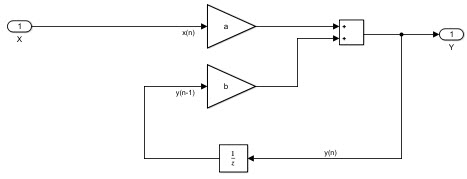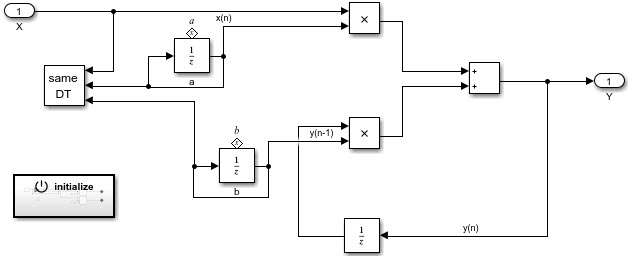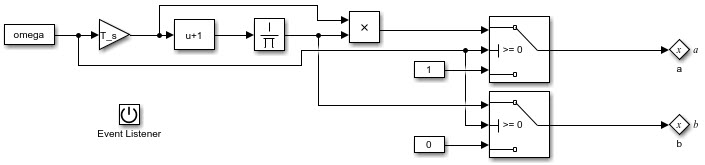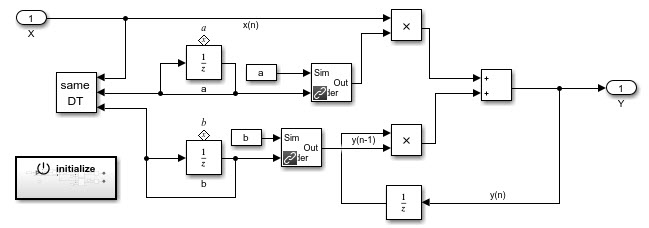Chinese Title: 为 Simulink Embedded Coder 增加模块中间变量的初始化功能

## Transfer Function of First-Order LPF

For a simulink block, the mask’s initialization commands is not included in the generated code. Take a first-order low-pass filter for example, the Laplace transfer function

{ Y(s) = \cfrac{\omega}{s+\omega}}

In the time domain,
{y_n = a \cdot x_n + b \cdot y_{n-1}}, where

a = \cfrac{\omega\cdot T_s}{1+\omega\cdot T_s}, \quad b=\cfrac{1}{1+\omega\cdot T_s}

and T_s is the sampling interval.

If we set \omega and T_s as mask parameters, we may want to calculate a and b in the mask initialization commands, i.e.

a = omega * T_s / ( 1 + omega * T_s );
b = 1 / ( 1 + omega * T_s );

and the block isHowever, when using code generation, the parameters a and b are embedded in the generated c or cpp code, rather than the expected parameters \omega and T_s. That’s because the mask initialization code is not included for coder.

## Improved Implementation with Parameter Initialization

There’s another approach, by using Initialize Function block. The filter block can be like thisCheck the Treat as atomic unit of the Low-Pass Filter block.

The Initialize Function block isBy this, the Embedded Coder will generate the initialization code calculating a and b from \omega and T_s. The initialization code should be called during initialization. After initialization, the code uses a and b as intermediate parameter to speed up low-pass filter calculation.

Note that, by using this approach, you will not be able to change the filter parameter during simulation (on the fly). The workaround is, try to use an Environment Controller block to separate coder and simulation, i.e.